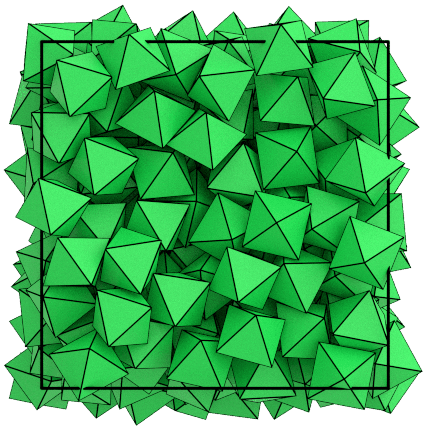# Performing Hard Particle Monte Carlo Simulations

## Overview

### Questions

• What is hard particle Monte Carlo?

• How do I set up a hard particle Monte Carlo simulation?

### Objectives

• Describe hard particle Monte Carlo simulations, particle shape, and trial moves.

• Show how to initialize the ConvexPolyhedron integrator.

• Explain the integrator parameters.

• Introduce time steps.

## Boilerplate code

:

import math

import hoomd


## Particle shape

A hard particle Monte Carlo (HPMC) simulation represents particles as extended objects which are not allowed to overlap. There are no attractive or repulsive forces in the system. The shape of the particle alone controls how it interacts with other particles. Formally, the potential energy of the system is zero when there are no overlaps and infinite when there are. Purely hard interactions induce effective attractions between particles which can lead to ordered structures. For example, hard regular octahedra will self-assemble into a bcc structure. In this tutorial, you will learn how to run a simulation of hard octahedra and observe this behavior.## The integrator

The ConvexPolyhedron integrator implements HPMC simulations - Create one:

:

mc = hoomd.hpmc.integrate.ConvexPolyhedron()


Set the shape property to define the particle shape. A convex polyhedron is defined by the convex hull of a set of vertices:

:

mc.shape['octahedron'] = dict(vertices=[
(-0.5, 0, 0),
(0.5, 0, 0),
(0, -0.5, 0),
(0, 0.5, 0),
(0, 0, -0.5),
(0, 0, 0.5),
])


## Trial moves

During each time step, HPMC attempts nselect trial moves on each particle in the system. Each trial move is drawn from a pseudorandom number stream and may be either a translation or rotation move. Translation moves displace a particle a random distance (up to d) in a random direction. Rotation moves rotate the particle by a random angle about a random axis. Larger values of a lead to larger possible rotation moves.

Any trial move whose shape overlaps with another particle is rejected, leaving the particle’s position and orientation unchanged. Any trial move whose shape does not overlap with any other particle is accepted, setting the particle’s position or orientation to the new value.

nselect, d, and a are properties of the integrator:

:

mc.nselect = 2
mc.d['octahedron'] = 0.15
mc.a['octahedron'] = 0.2


## Setting the integrator

:

cpu = hoomd.device.CPU()
sim = hoomd.Simulation(device=cpu, seed=1)


An integrator is a type of operation. There can only be one integrator in a Simulation and it operates on the system state on every time step. Assign the HPMC integrator to the Simulation to use it:

:

sim.operations.integrator = mc


The seed value (passed to the simulation constructor above) selects the sequence of values in the pseudorandom number stream. Given the same initial condition and seed, HPMC simulations will produce exactly the same results.

All operations that generate psuedorandom numbers use the seed set in the simulation. Whenever you add operations that utilize random numbers, you should set the seed to a non-default value.

Now you have a Simulation and a ConvexPolyhedron integrator, but can’t run the simulation yet. You first need to define the system state for the integrator to operate on. The next section in this tutorial will show you how to initialize the state.